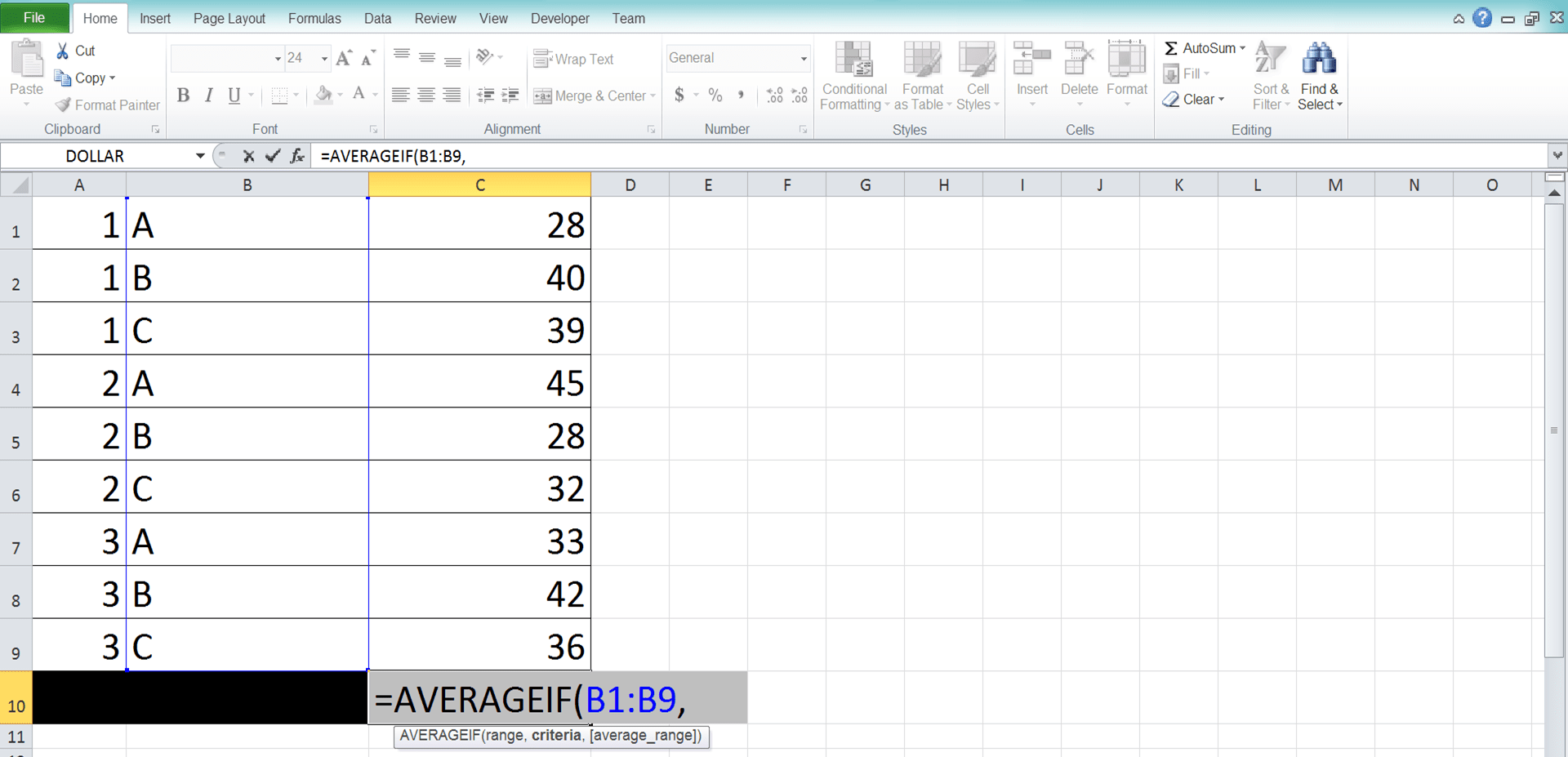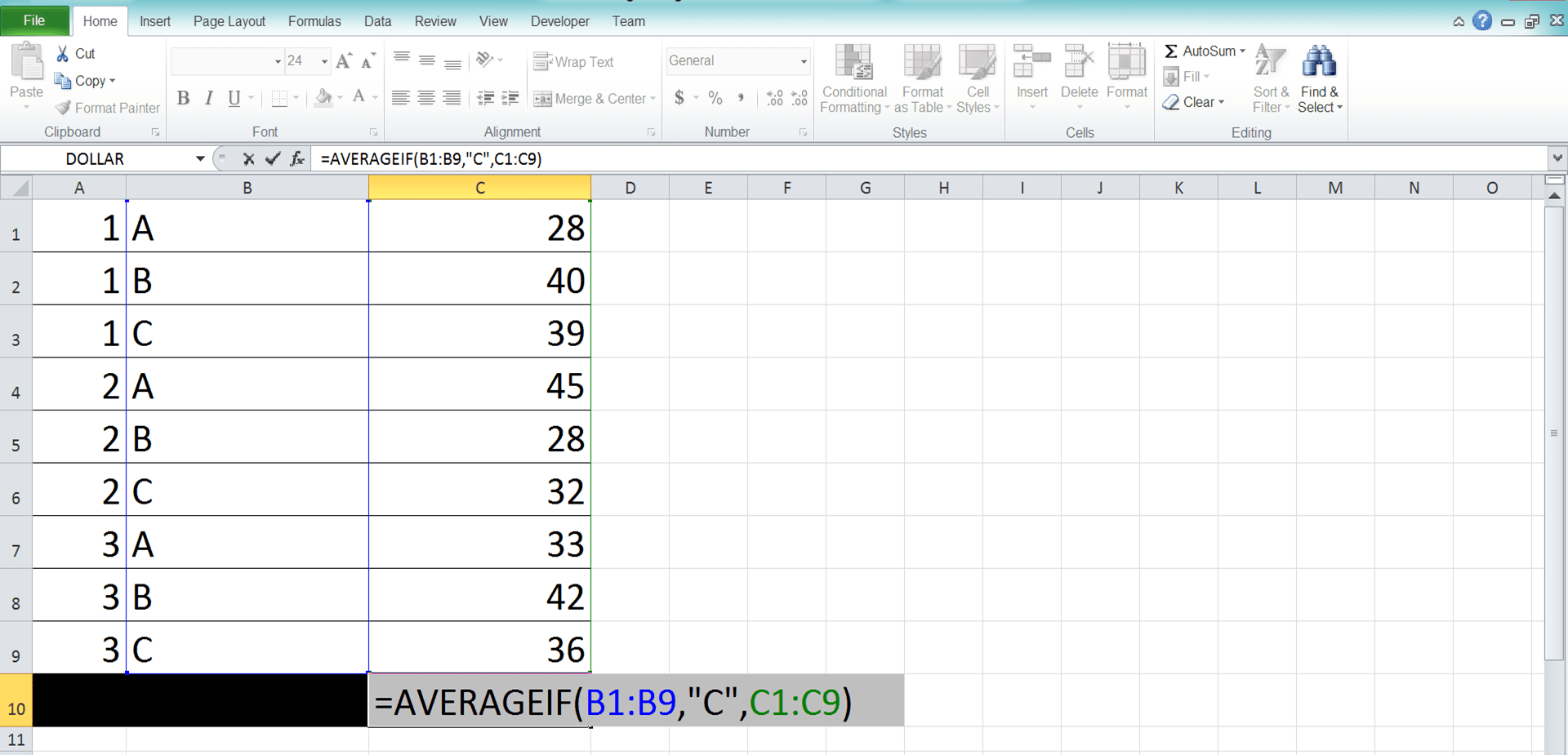How to Use the AVERAGEIF Function in Excel: Usabilities, Examples, and Writing Steps - Compute Expert

# How to Use the AVERAGEIF Function in Excel: Usabilities, Examples, and Writing Steps

Home >> Excel Tutorials from Compute Expert >> Excel Formulas List >> How to Use the AVERAGEIF Function in Excel: Usabilities, Examples, and Writing Steps

In this tutorial, you will learn how to use the AVERAGEIF function in excel completely.

When working with numbers in excel, we may sometimes need to average numbers from our data entries that fulfill a criterion. If we know how to use AVERAGEIF, then we can perform the average calculation fast and easily.

Want to know more about AVERAGEIF and master the way to use the function in excel? Read this tutorial until its last part!

Disclaimer: This post may contain affiliate links from which we earn commission from qualifying purchases/actions at no additional cost for you. Learn more

## What is the AVERAGEIF Function in Excel?

AVERAGEIF is an excel function that helps you to average numbers from data entries that fulfill a criterion.

## AVERAGEIF Usability

You can use AVERAGEIF to get the average of the numbers from data entries that fulfill your criterion.

## AVERAGEIF Result

The AVERAGEIF result is a number that represents the average of the numbers from selected data entries.

## Excel Version from Which We Can Start Using AVERAGEIF

We can start using AVERAGEIF in excel since excel 2007.

## The Way to Write It and Its Inputs

Here is the general writing form of AVERAGEIF in excel.

= AVERAGEIF ( data_range , criterion , [ number_range ] )

And here is a bit explanation of the inputs we need to give when we write an AVERAGEIF formula.
• data_range = the cell range (usually in the form of a column/row) that contains data from your data entries which you want to evaluate with your criterion. AVERAGEIF will only average the numbers from the data entries which data passes your criterion
• criterion = the criterion which evaluates the data in the cell range you inputted earlier
• [number_range] = optional. The cell range (usually in the form of a column/row) that contains the numbers you want to average from your data entries. This cell range should be parallel with and has the same size as the data cell range. If you don’t give input here, then AVERAGEIF will average the numbers in your data cell range

## Criterion Writing in AVERAGEIF

One of the most important things when writing an AVERAGEIF in excel is to get the criterion writing right. Wrong criterion writing can make your AVERAGEIF produces a wrong result or even an error!

Then, how do we write a criterion correctly in excel? Well, here are some examples of the right criterion writing in AVERAGEIF and their meanings. By following the writing pattern of these examples, you should be able to write your AVERAGEIF criterion correctly.

Text (not case-sensitive)
Criterion ExampleExplanation
"Jim"The same as “Jim”
“<>Jim”Not the same as “Jim”
“Jim*”With “Jim” prefix
“*jim”With “jim” suffix
“J*m”“J” prefix and “m” suffix
“Jim?”“Jim” prefix with any one character suffix
“?jim”Any one character prefix with “jim” suffix
“J?m”“J” prefix, any one character, and “m” suffix
“Jim~*”The same as “Jim*”
“Jim~?”The same as “Jim?”

A bit explanation of the symbols we use in some of the text criteria:
• * = any character with any amount
• ? = any one character
• ~ = use this when you want to add a literal * or ? character in the criterion

Number
Criterion ExampleExplanation
70Equal to 70
“>70”More than 70
“<70”Less than 70
“>=70”More than or equal to 70
“<=70”Less than or equal to 70

Date
Criterion ExampleExplanation
“>”&DATE(2019,12,3)Later than 3 December 2019

We don’t recommend inputting a date criterion through a direct writing method (e.g. “>3-12-2019”). It can produce a wrong and unexpected result for you.

Cell coordinate
Criterion ExampleExplanation
“>”&B1More than the value in B1

Empty/non-empty cell
Criterion ExampleExplanation
“"Empty
“<>"Not empty

## Example of Its Usage and Result

Below is a usage and result example of an AVERAGEIF formula in excelIn the example above, we want to calculate the average of the sales quantities from week 6. As we have one criterion for our average calculation here (from week 6), we can use AVERAGEIF to help us get the result.

As we discussed before, we need to input our data range (week column) and our criterion to AVERAGEIF. Since the data range and the number range (sales quantities column) aren’t the same here, we input the number range too.

The criterion we input here is “>=6”. It means we should get numbers which week is more than or equal to 6.

We input all of those to our AVERAGEIF and we get the sales quantities average that we want in the example (1495.8)!

## Writing Steps

After discussing the AVERAGEIF example, now let’s discuss how to write the AVERAGEIF formula from the beginning. We will discuss the writing steps with their implementation example screenshot too to help you learn them easier.

1. Type an equal sign ( = ) in the cell where you want to put your AVERAGEIF result2. Type AVERAGEIF (can be with large and small letters) and an open bracket sign after =3. Input the cell range (usually a column/row) which data you want to evaluate with your criterion. Then, type a comma sign ( , )5. Optional: Type a comma sign. Then, input the cell range (usually a column/row) where the numbers you want to average are. This cell range should be parallel with and has the same size as the cell range you input before

If you omit this input, then AVERAGEIF will average the numbers in the data cell range you input earlier6. Type a close bracket sign7. Press Enter
8. Done!## AVERAGEIF with a Not Blank Criterion

What if we want to average numbers from our data entries that aren’t blank in one of its columns/rows? What is the way to do this with an AVERAGEIF?

We can do that if we write “<>” in our AVERAGEIF criterion input part. Here is the general writing form of the AVERAGEIF with the criterion input.

= AVERAGEIF ( data_range , “<>” , [ number_range ] )

Simple, isn’t it? And here is an implementation example of the formula writing in excel.In this example, we want to average the sales quantities which number have been approved. The approved sales quantities have an “approved” mark in the “Approved?” column while the non-approved” ones are blank there.

To calculate the average fast and easily, we use AVERAGEIF with a not blank criterion. As we discussed before, we need to input “<>” to represent the not blank criterion in the AVERAGEIF.

As a result of that, we get the approved sales quantities average that we want here (1668.6)!

## AVERAGEIF with a Not 0 Criterion

Besides blank, we may sometimes want to calculate the average of our numbers in excel with a not 0 criterion. This is usually because we don’t want the zeroes we have to make our average calculation result wrong.

To do that by using AVERAGEIF, we need to input “<>0” as our criterion input in the formula. Here is the general writing form of the AVERAGEIF with the criterion input.

= AVERAGEIF ( data_range , “<>0” , [ number_range ] )

And here is its implementation example in excel.If you want the criterion to be more than zero instead (maybe you want to avoid the negative numbers too), you need to input “>0” for your AVERAGEIF criterion.

## AVERAGEIF with Multiple Criteria: AVERAGEIFS

Need to evaluate multiple criteria for your average calculation process? For this, you should use the AVERAGEIF sibling formula instead, AVERAGEIFS.

AVERAGEIFS is an excel formula that can also help you to average numbers from your data entries that fulfill your criteria. It can accept more than one criteria, unlike AVERAGEIF which can only accept one.

The general writing form of AVERAGEIFS is like this in excel.

= AVERAGEIFS ( number_range , data_range1 , criterion1 , … )

Different from AVERAGEIF, you must input a number range in AVERAGEIFS and you must input it first. After you input the number range, you can input your pairs of data range and criterion until 127 pairs. You should input as many as the criteria you have in your average calculation.

AVERAGEIFS will only calculate the average of the numbers from data entries that fulfill all your criteria. Thus, it will serve our purpose in calculating an average result with multiple criteria!

Here is an implementation example of AVERAGEIFS in excel to make you understand the formula concept easier.In this example, we have from week 2, apple product, and region B as our sales quantities average calculation criteria. Our sales quantities must fulfill all those criteria before we can average them.

As we have multiple criteria for our average calculation process here, we use AVERAGEIFS. We input the sales quantity column and the pairs of the week, product, and region column and criterion to it.

By doing that, we immediately get the average calculation result that we need!

## Exercise

After you learned how to use the AVERAGEIF function in excel, now let’s practice your understanding by doing the exercise below!

### Questions

1. What is the average production amount from machine A in the period?
2. What is the average reliability of machine D in the period?
3. What is the average production hours from machine C in the period?

AVERAGEIFS can also process your average calculation if you only have one criterion. Thus, you might not need to use AVERAGEIF anymore if you have already mastered AVERAGEIFS.

Related tutorials you should learn:

Get updated excel info from Compute Expert by registering your email. It's free!